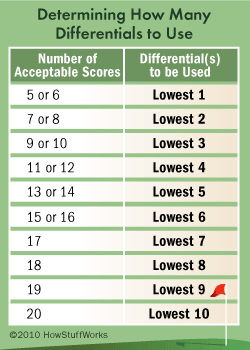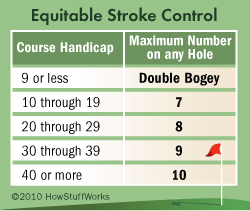# How to Calculate Your Golf Handicap

## Understanding Golf HandicapsCalculating a golf handicap can seem confusing at first. But once you understand the basics, all you'll need is a grasp of elementary school arithmetic. The first thing you should know is the difference between a scratch golfer, whose handicap is zero, and a bogey golfer, whose handicap is 18. These terms are important because they influence two very important variables: course rating and slope rating. The course rating is typically a number between 67 and 77 that approximates the score of a scratch golfer. A course's slope rating is a number between 55 and 155 that indicates the course difficulty for a bogey golfer relative to a scratch golfer. Both ratings should be found on your golf scorecard.

Finally, you need to know how to find your adjusted gross score. Also known as an equitable stroke control (ESC) score, this variable sets a limit on the number of strokes you have to count on an exceptionally bad hole. The lower your handicap, the fewer strokes you have to count. If you haven't been assigned a handicap, you'll have to count the maximum allowed: up to 10 strokes per hole. After adjusting your score for each hole, you can add them all together to get your adjusted gross score.

Once you have all of this information, you can calculate your handicap differential for each round. To do this, subtract the course rating from your adjusted gross score. Then multiply this number by 113 and divide by the slope rating. In other words:

handicap differential = (adjusted gross score - course rating) x 113 / slope rating

Differentials are rounded to the nearest tenth.The next step is to calculate your handicap index. If you've played 20 rounds of golf on any USGA rated course, you'll take the average of your lowest 10 handicap differentials and multiply the average by 0.96. Any numbers after the tenth digit can be deleted. You can calculate your handicap index after playing as few as five rounds, but the more rounds you play, the more accurate your index will be.

You can then use your handicap index to calculate your course handicap for a specific course. Simply multiply your handicap index by the slope rating and divide by 113. Round the result to the nearest whole number:

course handicap = (handicap index x slope rating) / 113

When you finish a round of golf, you can subtract your course handicap from adjusted gross score for that round to get your net score.

net score = adjusted gross score - course handicap

If all this is still a little unclear, read on to see a sample handicap calculation.